# CLASS 11 MATHS CHAPTER-9 SEQUENCES AND SERIES

### Write the first five terms of each of the sequences in Exercises 1 to 6 whose nth terms are:

Question1. Write the first five terms of the sequences whose nth term is an=n(n+2).

Solution :
an=n(n+2)

Substituting n = 1, 2, 3, 4, and 5, we obtain

a1=1(1 + 2)=3

a2=2(2 + 2)=8

a3=3(3 + 2)=15

a4=4(4 + 2)=24

a5=5(5 + 2)=35

Therefore, the required terms are 3, 8, 15, 24, and 35.

Question2. Write the first five terms of the sequences whose nth term is a n= n/n+1.

Solution :
Given: n= n/n+1.

Putting n = 1 and 5, we get,

Question3.  an = 2n

Solution :
Given:  nth term ,  an = 2n

Putting n = 1,2,3,4,and 5, we get,

a1 = 21 = 2

a2 = 22 = 4

a3 = 23 = 8

a4 = 2= 16

a5 = 2= 32

Therefore, the first five terms are 2, 4, 8, 16 and 32.

Question4. an = (2n-3) / 6

Solution :
Given: an = (2n-3) / 6

Putting 1and 5, we get,

Therefore, the first five terms are -1/6, 1/6 , 1/2 , 5/6 and 7/6

Question5. a= (−1)n−1 5n+1

Solution :
Given:  = (−1)n−1 5n+1

Therefore, the first five terms are 25, -125, 625, -3125 and 15625

Question6.  Write the first five terms of the sequences whose nth
term is

Solution :
Given:

Putting n = 1 2,3,4 and 5, we get,

Find the indicated terms in each of the sequences in Exercises 7 to 10 where 17th terms are:

Question7. an=4n−3;  a17, a24

Solution :
Given: The given equation is an=4n−3

.

Substitute n=17

in the equation.

a17=4(17)−3

⇒a17=65

Similarly substitute n=24

in the equation.

a24=4(24)−3

⇒a24=93

Therefore, the 17th
and 24th
term of an=4n−3
is 65
and 93
respectively.

Question8. an = n2 / 2n ;a7

Solution :
Given:

The given equation is a= n2  /  2n

.

Substitute n = 7 in the equation.

a7  =  7227

⇒a7  =  49128

Therefore, the 7th
term of an=n /  2n
is 49128

.

Question9. an=(−1)n−1n3 ;a9

Solution :
Given:

The given equation is an=(−1)n−1n3

Substitute n=9

in the equation.

a9  =   (−1)9−193

⇒a9  =  729

Question10.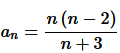Solution :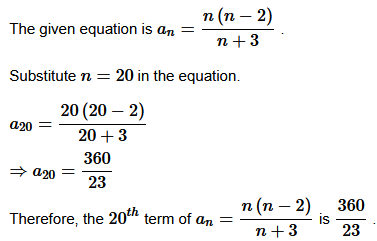Write the first five terms of each of the sequences in Exercises 11 to 13 and obtain the corresponding series:

Question11. a1 =3, an = 3an-1 + 2 for all n > 1

Solution :
Given:  a1 =3, an = 3an-1 + 2 for all n > 1

Putting 1 ,2,3,4 and 5, we get

The given equation is an  =  3an−1+2
where a1 = 3
and n>1

.

Substitute n=2
and a1 = 3

in the equation.

a2  = 3a2 − 1+2 =3(3) + 2

⇒ a2 =11

Similarly substitute n=3,4
and 5

in the equation.

a3 = 3a− 1 + 2=3(11)+2

⇒a3 = 35

a4 = 3a4 − 1+2 = 3(35)+2

⇒a= 107

a5 = 3a5−1 + 2 = 3(107)+2

⇒a= 323

Therefore, the first five terms of an=3an−1+2
is 3,11,35,107
and 323

.

Question12. Write the first five terms of the following sequence and obtain the corresponding series:

a1= −1,a= an−1 / n, n≥2

Solution :
Given: a1= −1,a= an−1 / n, n≥2

Question13. Write the first five terms of the following sequence and obtain the corresponding series:

a1 = a2 = 2, an = an−1 −1, n>2

Solution :
Given:

The given equation is a= an−1 −1
where a1= a=2
and n>2

.

Substitute n=3
and a2 = 2

in the equation.

a3=a3−1 − 1 = 2−1

⇒a3 = 1

Similarly substitute n=4
and 5

in the equation.

a4 = a4−1−1 = 1−1

⇒a4 = 0

a = a5−1 −1 =  0−1

⇒a= −1

Therefore, the first five terms of a= an−1 −1
is 2,2,1,0
and −1

.

The corresponding series obtained from the sequence is 2+2+1+0+(−1)+…
.

Question14. The Fibonacci sequence is defined by  1= a1= a2 and an= an−1 + an−2, n>2 Find an+1/ an, for n=1, 2, 3, 4, 5

Solution :
Given: 1= a1= a2 and an= an−1 + an−2

Putting 1,2,3, 4, 5 and 6, we have

The given equation is an=an−1−1
where a1=a2=2
and n>2

.

Substitute n=3
and a2=2

in the equation.

a3=a3−1−1=2−1

⇒a3=1

Similarly substitute n=4
and 5

in the equation.

a4=a4−1−1=1−1

⇒a4=0

a5=a5−1−1=0−1

⇒a5=−1

Therefore, the first five terms of an=an−1−1
is 2,2,1,0
and −1

Exercise 9.2

Write the first five terms of each of the sequences in Exercises 1 to 6 whose nth terms are:

Question 1. Find the sum of odd integers from 1 to 2001.

Solution :

Odd integers from 1 to 2001 are 1, 3, 5, 7, …….., 2001.

The first term of the A.P. is a=1
and the common difference is d=2

.

The nth
term of the A.P. is given by the equation an=a+(n−1)d

.

Therefore, a+(n−1)d=2001

Substitute a=1
and d=2

in the equation.

⇒1+(n−1)2=2001

⇒2n−1=2001

⇒n=2001+1  /  2

⇒n=1001

The sum of first n
terms of an arithmetic progression is given by the equation

.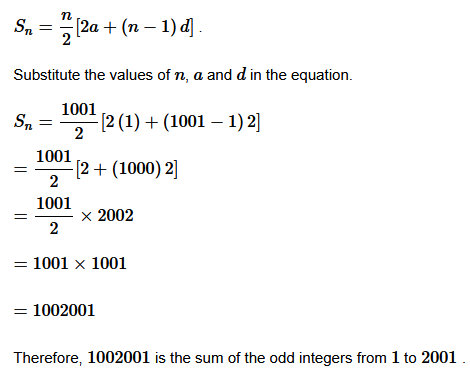Question 2. Find the sum of all natural numbers lying between 100 and 1000 which are multiples of 5.

Solution :

105,110,…,990,995 are the natural numbers lying between 100
and 1000
, which are multiples of 5

. An A.P. is formed by this sequence.

The first term of the A.P. is a=105
and the common difference is d=5

.

The nth
term of the A.P. is given by the equation an=a+(n−1)d

.

Therefore, a+(n−1)d=995

Substitute a=105
and d=5

in the equation.

⇒105+(n−1)5=995

⇒5n+100=995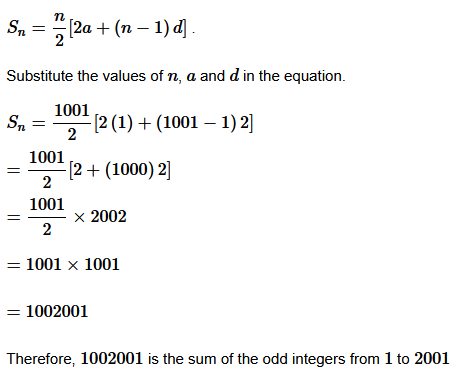Question 3. In an A.P. the first term is 2 and the sum of the first five terms is one-fourth of the next five terms. Show that 20th term is -112.

Solution :

The first term of the A.P. is 2
and let the common difference of the A.P. be d

.

Then 2,2+d,2+2d,2+3d,…

is the A.P.

10+10d
is the sum of the first five terms and 10+35d

is the sum of the next five terms.

According to the conditions given in the question,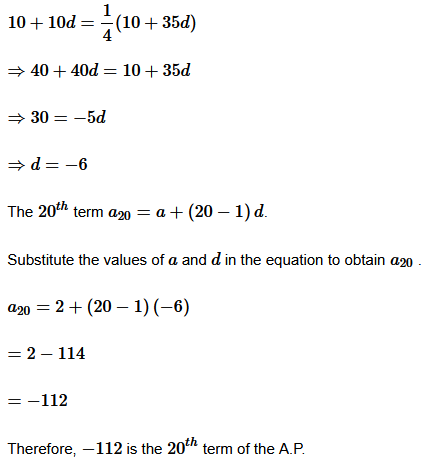Question 4. How many terms of the A.P., -6, -11/2 ,-5 ……………. are needed to give the sum -25.?

Solution :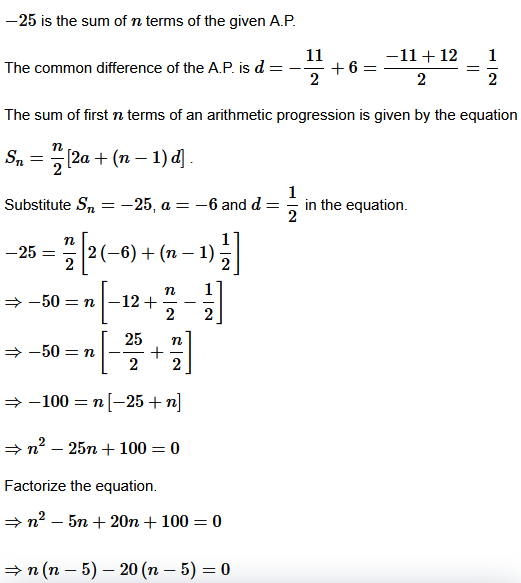⇒ n = 20 ,5

There for  , 5 or 20 terms of the Ap are needed to give he sum  – 25 .

Question 5. In an A.P., if pth term is 1/q  and qth term is 1/p , prove that the sum of first pq terms is 1/2(pq+1),  where  p≠q

Solution :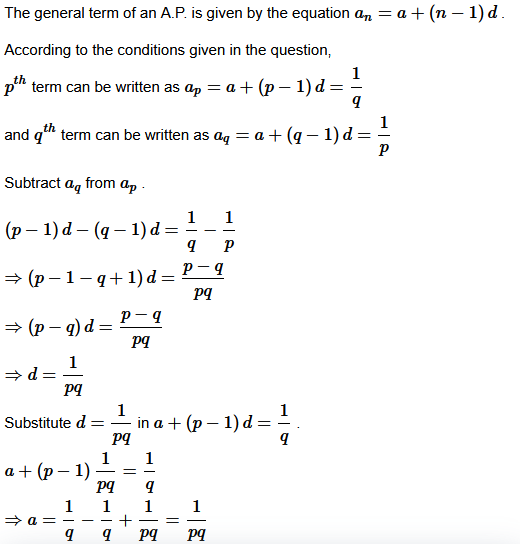The sum of first n terms of an arithmetic progression is given by the equation

Question 6. If the sum of a certain number of terms of the A.P. 25, 22, 19, ….. is 116, find the last term.

Solution :
Here116 is the sum of n

terms of the given A.P.

The common difference of the A.P. is d=22−25=−3

The sum of first n
terms of an arithmetic progression is given by the equatio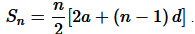Substitute Sn=116
, a=25
and d=−3

in the equation.

116=n / 2[2(25)+(n−1)−3]

⇒232=n[50+−3n+3]

⇒232=n(53−3n)

⇒3n2 −53n+232=0

Factorize the equation.

⇒3n2−24n−29n+232=0

⇒3n(n−8)−29(n−8)=0

⇒(n−8)(3n−29)=0

⇒n=8 or 29 /3

n=8 as n cannot be equal to 29 / 3

As n=8 the last term is

a= a + (8−1)d

Substitute a=25 and d=−3

in the equation.

a8 = 25+(7)(−3)

=25−21

=4

Therefore, 4 is the last term of the A.P.

Question 7. Find the sum to n terms of the A.P., whose kth term is 5k + 1.

Solution :
5k+1 is given as the kth term of the A.P. The equation for kth
term of an A.P. is given as ak+(k−1)d

.Then,

a+(k−1)d = 5k + 1

⇒a + kd − d = 5k + 1

By comparing the coefficient of k we get the value of d as 5

⇒a−d=1

⇒a−5=1

⇒a=6

The sum of first n
terms of an arithmetic progression is given by the equation

Question 8. If the sum of n terms of an A.P. is (pn + qn2) where p and q are constants,find the common difference.

Solution :
Given: (pn + qn2)

The sum of first n terms of an arithmetic progression is given by the equation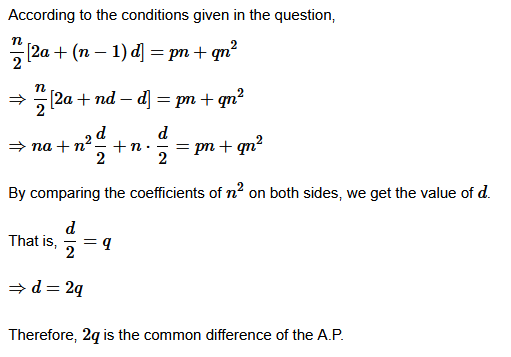Question 9. The sums of n terms of two arithmetic progressions are in the ratio 5n + 4 : 9n + 6. Find the ratio of their 18th  terms.

Solution :
Let a1 and d1 be the first term and the common difference of the first arithmetic progression respectively and a2 and d2 be the first term and the common difference of the second arithmetic progression respectively.
hbAccording to the conditions given in the question, Sum of n terms of first A.P / Sum of n terms of second A.P.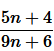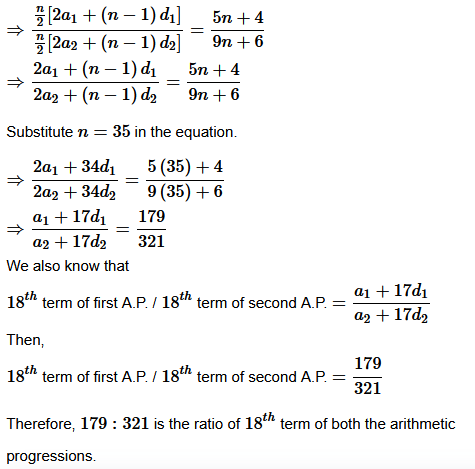Therefore, the ratio of 18th terms of two A.P.’s is 179: 321.
Question 10. If the sum of first p terms of an A.P. is equal to the sum of the first q terms, then find the sum of the first (p + q) terms.

Solution :

Let a
be the first term and d

be the common difference of the A.P.

The sum of first p
terms of the A.P. is given by the equation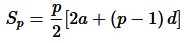and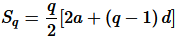= P+q / 2[2a -2a ]

= 0

= Therefore, 0 is the sum of the first (p+q) terms of the A.P.

Question 11. Sum of the first p, q and r terms of an A.P. are a, b and c, respectively. Prove that a/p (q−r) + b/q(r−p) + c/r(p−q) = 0.

Solution :
Let A be the first term and d be the common difference of given A.P.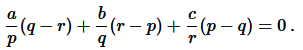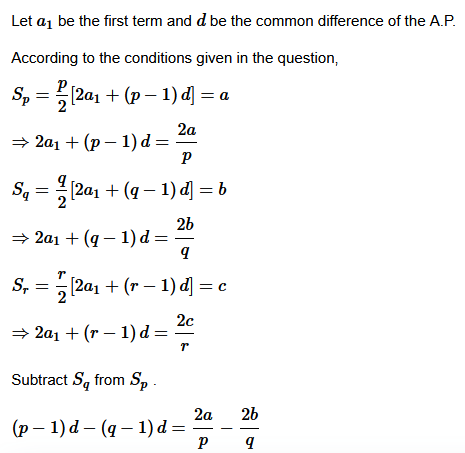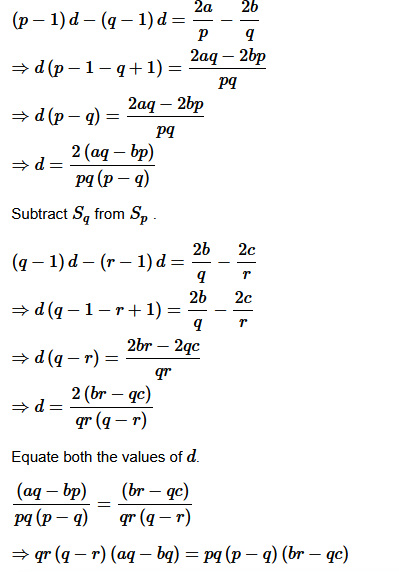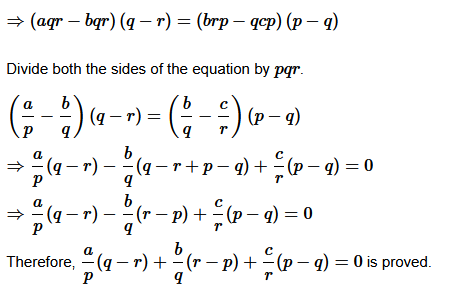L.H.S. = R.H.S. Proved.
Question 12. The ratio of the sums of m and n terms of an A.P. is m2 : n2 . Show that the ratio of mth  and nth  term is (2m – 1) : (2n – 1).

Solution :
Let a be the first term and d be the common difference of given A.P.
Let a be the first term of the A.P. and d be the common difference. According to the conditions given in the question, Sum of m terms / Sum of n termsand  m/ n2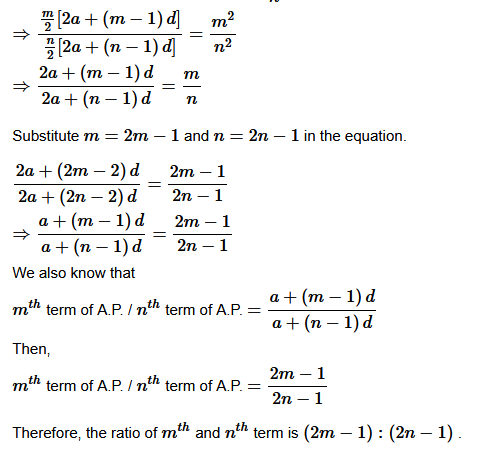Question 13.If the sum of n terms of an A.P. is 3n+ 5n and its mth term is 164, find the value of m.

Solution :
: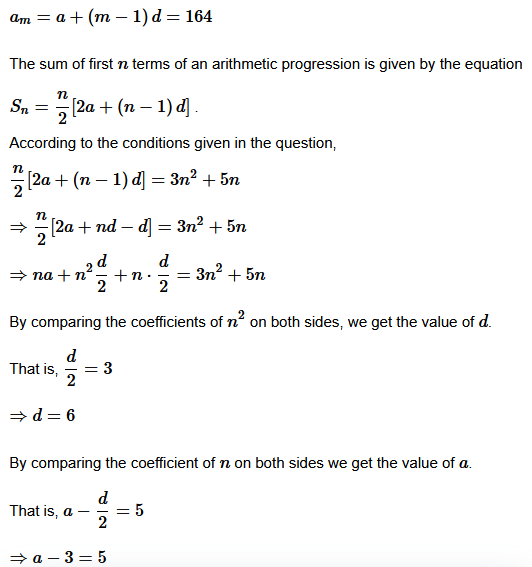Question 14. Insert five numbers between 8 and 26 so that the resulting sequence is an A.P.

Solution :
Let the five numbers between 8
and 26
be A1,A2,A3,A4 and A5
. Then the resulting sequence 8,A1,A2,A3,A4,A5,26

is an A.P.

The first term of the A.P. a=8
, the last term b=26
and the number of terms n=7

.

Substitute the values of a,b
and n
in b=a+(n−1)d

.

⇒26=8+(7−1)d

⇒6d=26−8

⇒d=3

Then substituting the values of a
and d

we get

A1=a+d=8+3=11

A2=a+2d=8+2(3)=14

A3=a+3d=8+3(3)=17

A4=a+4d=8+4(3)=20

A5=a+5d=8+5(3)=23

Therefore, 11,14,17,20
and 23
are the five numbers between 8
and 26
Question 15. If is the A.M. between a and b then find the value of n.

Solution :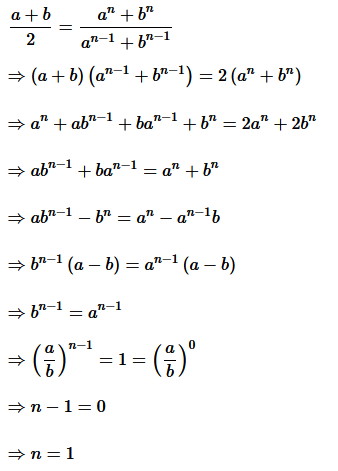Question 16. Between 1 and 31, m numbers have been inserted in such a way that the resulting sequence is an A. P. and the ratio of 7th and (m–1)th  numbers is 5 : 9. Find the value of m.

Solution :
Let A. P a1, A2, A3, A4, …….., Am be  n = m + 2 numbers between 1 and 31.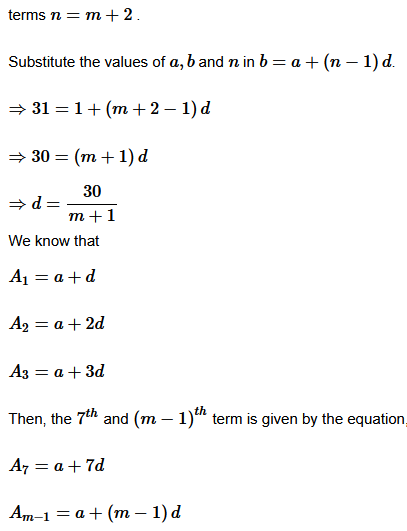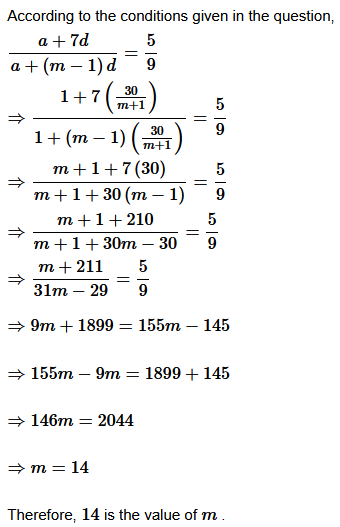Question 17. A man starts repaying a loan as first installment of Rs. 100. If he increases the installment by Rs. 5 every month, what amount he will pay in the 30th installment?

Solution :

Amount of 1st installment = Rs. 100 and Amount of 2nd installment = Rs. 105
Rs.100
is the first installment of the load and Rs.105

is the second installment and so on.

Therefore, an A.P. is formed by the amount that the man repays every month.

100,105,110…

is the A.P.

The first term of the A.P. a=100
and the common difference d=5
The 30th  is given by the equation A30=a+(30−1)d

.

⇒A30 = 100+(29)5

=100+145

=245

Therefore, Rs.245
is the amount to be paid in the 30th
installment.= Rs. 245
Therefore, the amount of 30th installment is Rs. 245.
Question 18. The difference between any two consecutive interior angles of a polygon is 5º If the smallest angle is 120º find the number of the sides of the polygon.

Solution :

An A.P. is formed by the angles of the polygon. The first term of the A.P. a = 120
and the common difference d = 5

.

We know that 180(n−2)
is the sum of all angles of a polygon with n

sides.

Therefore, S= 180(n−2)

⇒n/2 [2a+(n−1)d] = 180(n−2)

⇒n/2[240+(n−1)5] = 180(n−2)

⇒n[240+(n−1)5] = 360(n−2)

⇒240n+5n2−5n=360n−720

⇒5n−125n+720=0

⇒n− 25n + 144=0

⇒n2 − 16n−9n+144=0

⇒n(n−16)−9(n−16)=0

⇒(n−9)(n−16)=0

⇒n=9
or 16

Therefore, the number of the sides of the polygon 9
or 16

Exercise 9.3
Question1. Find the 20th and nth terms of the G.P. 5/2,5/4,5/8

Solution :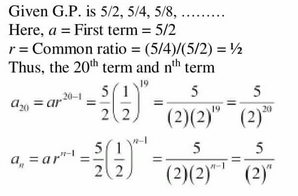Question2. Find the 12th term of a G.P. whose 8th term is 192 and the common ratio if 2.

given

common ratio of G.P  r = 2;

Let a be the first term of G.P

Solution :

given common ratio of G.P r = 2; Let a be the first term of G.P

Question3. The 5th,8th and 11th terms of a G.P. are p, q and s, respectively.  Show that q2=ps.

Solution :

Let a be the first term and r be the comman ratio of G.P according to given condition

Dividing Equation (2) by (1) We obtained

Dividing Equation (3) by (2) We obtained

Equating the equation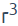obtained in (4) and (5) we obtain

Thus given result is proved

Question 4. The 4th term of a G.P. is square of its second term and the first term is -3. Determine its 7th term.

Solution :
Let

Acording to the Question

Question 5. Which term of the following sequences:
(a) 2 ,2√2,4,… is 128?
(b) √3, 3, 3√3,….is 729?
(c) 1/3, 1/9, 1/27,… is 1/19683?

Solution :

Therefore, 13th term of the given G.P. is 128.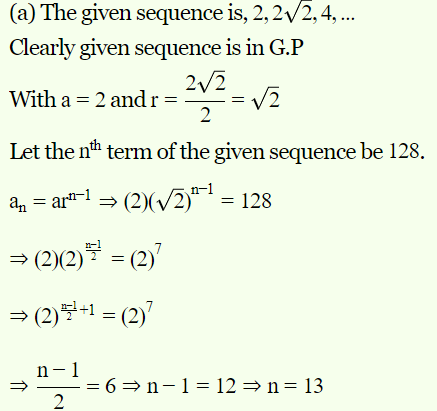Therefore, 12th term of the given G.P. is 729.

Question 6. For what values of x, the numbers −2/7,x,−7/2a are in G.P.?

Solution :
Given: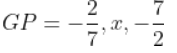are in G.P.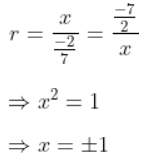Thus, for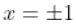given noumbers will be  i Gp

Find the sum to indicated number of terms in each of the geometric progression in Exercises 7 to 10:
Question 7. 0.15, 0.015, 0.0015, ………. 20 terms

Solution :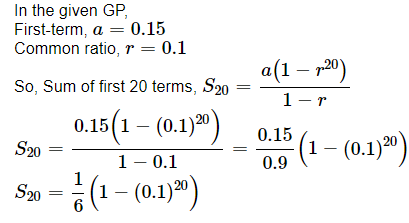Question 8. √7, √21, 3√7,…,n terms

Solution :
Here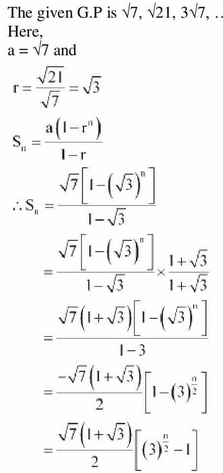Question 9.  Find the sum to n terms in the geometric progression 1,−a,  a2, −a3 … (  if  a≠ −1)

Solution :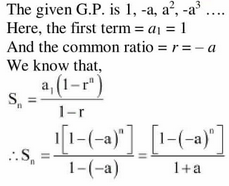Question 10. Find the sum to n terms in the geometric progression x3, x5,x,…n terms (if x≠±1)

Solution :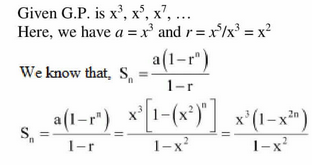Question 11. Evaluate: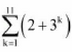Solution :
Given:

Question 12. The sum of first three terms of a G.P. is 39/10 and their product is 1.  Find the common ratio and the terms.

Solution :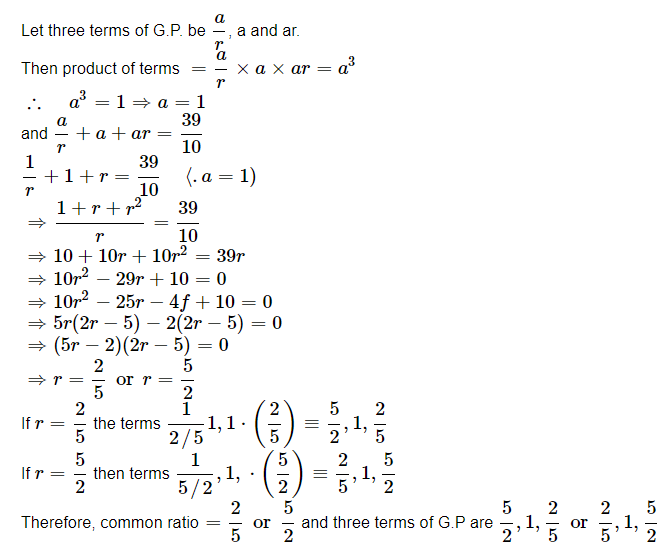Question 13. How many terms of G.P. 3,32,33,… are needed to give the sum 120?

Solution :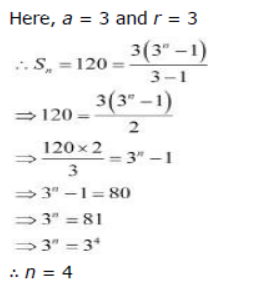Question 14. The sum of first three terms of a G.P. is 16 and the sum of the next three terms is 128.
Determine the first term, the common ratio and the sum to n terms of the G.P.

Solution :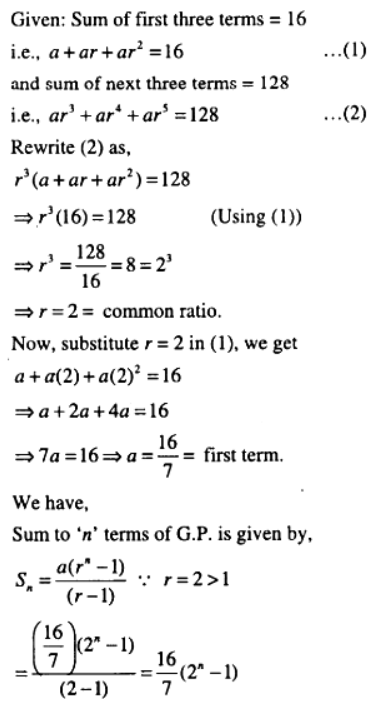Question 15. Given a G.P. with a= 729 and 7th term 64, determine S7;

Solution :
Given: a = 729 and a7 =64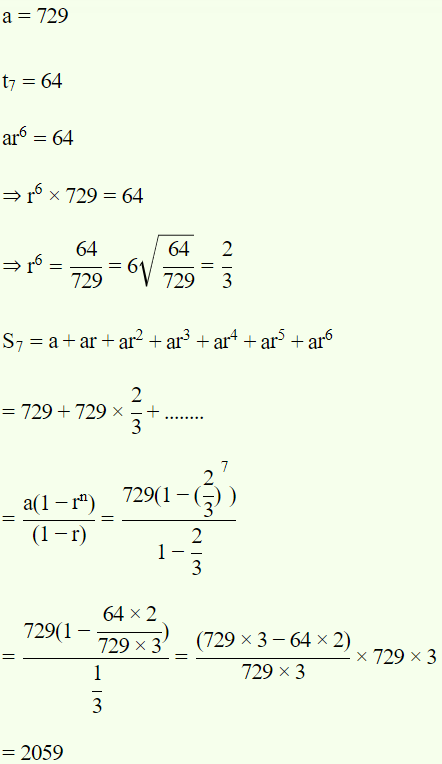Question 16. Find a G.P. for which sum of the first two terms is -4 and the fifth term is 4 times the third term.

Solution :

Given: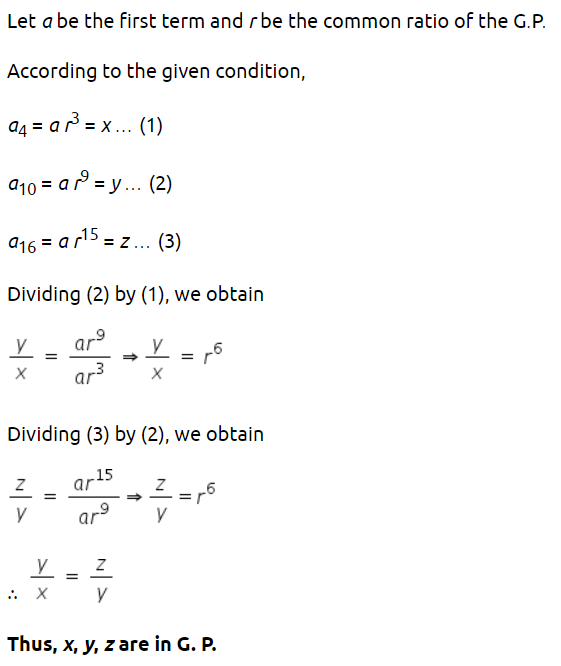Question 17. If the 4th,10thand16th terms of a G.P. are x, y and z, respectively.
Prove that x, y, z are in G.P.

Solution :Question 18. Find the sum to n terms of the sequences 8, 88, 888, 8888, ……

Solution :
Here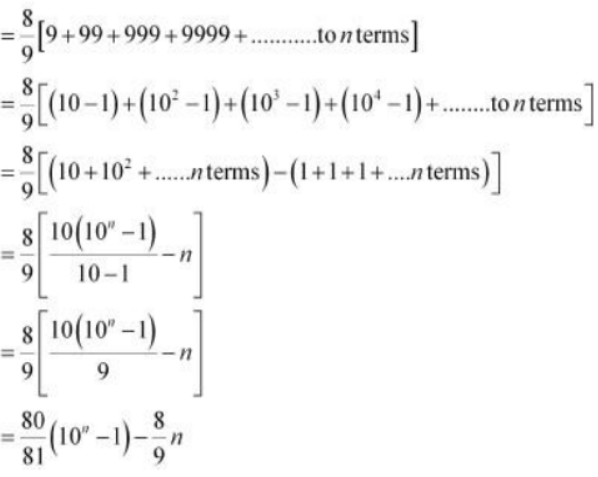Question 19. Find the sum of the product of the corresponding terms of the sequences 2, 4, 16, 32 and 128, 32, 8, 2, 1/2.

Solution :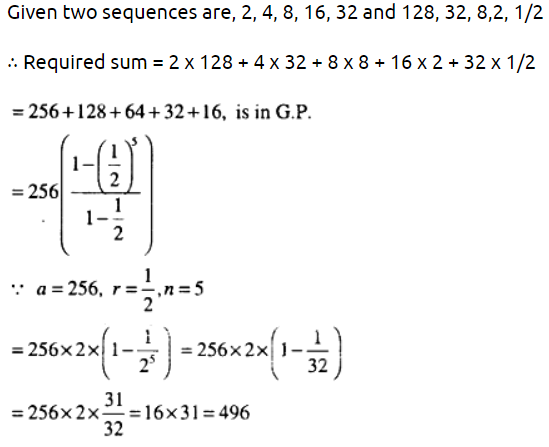Question 20. Show that the products of the corresponding terms of the sequences  a, ar, ar …arn−1 and A,AR, AR2,…ARn−1  form a G.P, and find the common ratio.

Solution :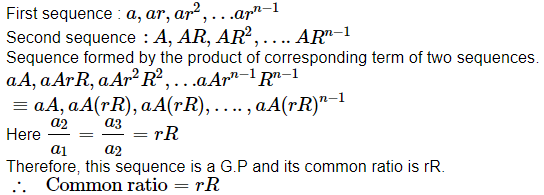Question 21. Find four numbers forming a geometric progression in which the third term is greater than the first term by 9 and the second term is greater than by 4th by 18.

Solution :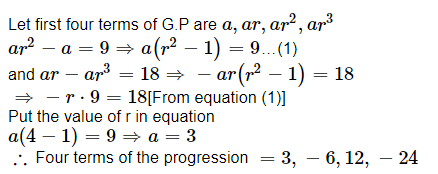Question 22. If the pth,qth and rth terms of a G.P. are a, b and c, respectively.  Prove that aq−r br−p cP−q =1

Solution :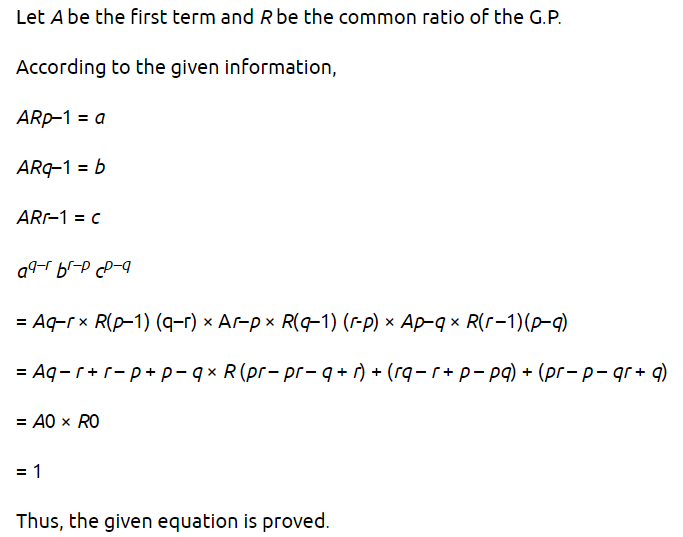Question 23. If the first and the nth term of a G.P. are a and b, respectively, and if P is the product of n terms, prove that  P2= (ab)n.

Solution :

The first term of the G.P is a  and the last term is b.

Therefore, the G.P. is a,ar,ar2 ,ar3…..arn-1, where r  is the common ratio.

b = arn-1   ….(1)

P = Product of n terms

= (a) (ar) (ar2) (ar3)…..(arn-1)

=(a x a x….a)(r x r² x…..rn-1)

= an r1 + 2 +(n-1)  …(2)

Here, 1, 2, …(n-1)  is an A.P.

Hence, proved

Question 24. Show that the ratio of the sum of first n terms of a G.P. to the sum of terms from (n+1) th  to (2n) th term is 1/ rn

Solution :

Question 25. If a, b, c and d are in G.P. show that (a2 + b2 + c2) (b2 + c2 + d2) = (ab + bc + cd)2

Solution :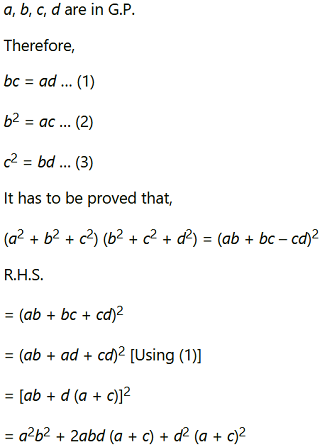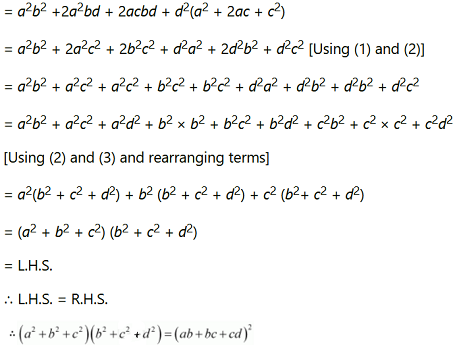Therefore, L.H.S. = R.H.S.

Question 26. Insert two numbers between 3 and 81 so that the resulting sequence us G.P.

Solution :
Let G1 and G2 be two numbers between 3 and 81 such that 3,G1 ,G2, 81 are in G.P.

Let a be the first term and r  be the common ratio of the G.P.

∴81 = (3)(r)3
⇒ r³ = 27

⇒ r = 3 (Taking real roots only)
For r = 3,

G1 = ar = (3)(3) = 9

G1 = ar2 = (3)(3)2 = 27

Thus, the required two numbers are 9 and 27

Question 27. Find the value of n so that may be the geometric mean between a and  b.

Solution :

Question 28. The sum of two numbers is 6 times their geometric mean, show that numbers are in the ratio ((3+2√2) : (3−2√2)

Solution :

Question 29. If A and G be A.M. and G.M. respectively between two positive numbers, prove that the numbers are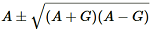Solution :

Question 30. The number of bacteria in a certain culture doubles every hour. If there were 30 bacteria present in the culture originally, how many bacteria will be present at the end of 2nd hour, 4th hour and nth hour?

Solution :
Bacteria present in the culture originally = 30
Since the bacteria doubles itself after each hour, then the sequence of bacteria after each hour is a G.P.
Here a = 30 and r = 2
∴ a3 = ar² = (30)(2)² =120

Therefore, the number of bacteria at the end of 2nd hour will be 120

a5 = ar4 = (30)(2)4 = 480

an+1 = arn = (30) 2n

Thus, number of bacteria at the end of nth hour will be (30) 2n

Question 31. What will Rs. 500 amount to 10 years after its deposit in a bank which pays annual interest rate of 10% compounded annually?

Solution :
The amount deposited in the bank is Rs 500.

At the end of first year, amount = Rs 500(1 +   1/10)= Rs 500 (1.1)

At the end of 2nd  year, amount = Rs 500 (1.1) (1.1)

At the end of 3rd  year, amount = Rs 500 (1.1) (1.1) (1.1) and so on

∴Amount at the end of 10 years = Rs 500 (1.1) (1.1) … (10 times)

= Rs 500(1.1)10

Question 32. If A.M. and G.M. of roots of a quadratic equation are 8 and 5 respectively then obtain the quadratic equation.

Solution :
Let a and b be the roots of required quadratic equation.
Then A.M. =  a+b/2 = 8 ⇒ a+b = 16      ….(1)

And G.M. = √ab = 5 ⇒ 25     …….(2)
Now, Quadratic equation is given by,

x2 – x (Sum of roots) + (Product of roots) = 0

x2 – x (a+b) + (ab) = 0

x– 16x + 25 = 0   [Using (1) and (2)]

Thus, the required quadratic equation is x– 16x + 25 = 0.

### Find the sum to n terms in each of the series in Exercises 1 to 7.

Question1. Find the sum to n terms of the series 1 × 2 + 2 × 3 + 3 × 4 + 4 × 5 +…

Solution :
Given:1 × 2 + 2 × 3 + 3 × 4 + 4 × 5 +…to nth terms

The nth term of the series is an = n(n+1)

Question2. Find the sum to n terms of the series 1 × 2 × 3 + 2 × 3 × 4 + 3 × 4 × 5 + …

Solution :

an =n(n+1)(n+2)

=(n+ n)(n + 2)

=n3 + 3n+ 2n
The sum of n terms of a series is given by the equation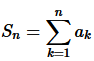=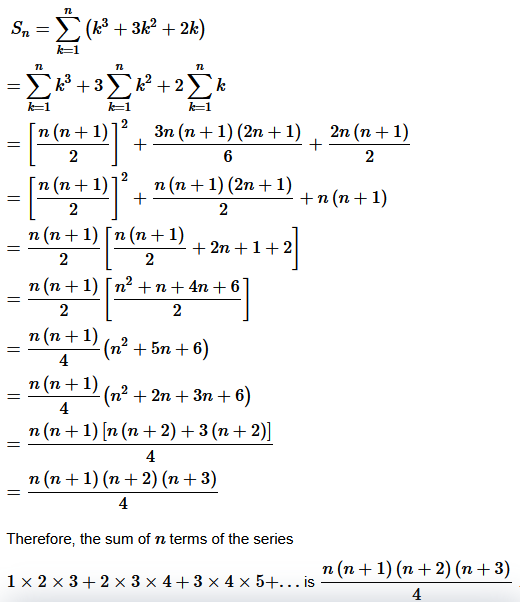Question3. Find the sum to n terms of the series 3 × 12 + 5 × 22 + 7×32+…

Solution :
Given: 3 × 12 + 5 × 22 + 7×32+…to   nth terms

Question4.

Solution :
Given:to nth terms

Question5. Find the sum to n terms of the series 52 + 62 + 72+…+202

Solution :

Given: 52 + 62 + 72+…+202

= The nth term of the series is

= an=(n+4)2

=n2+8n+16

= The sum of n terms of a series is given by the equationQuestion6. Find the sum to n terms of the series 3 × 8 + 6 × 11 + 9 × 14 + …

Solution :
Given: 3 × 8 + 6 × 11 + 9 × 14 + …to nth terms

an= (nth
term of 3,6,9…

(nth
term of 8,11,14…

)

=(3n)(3n+5)

=9n2+15n

The sum of n terms of a series is given by the equationThe sum of  n terms of the given series is
.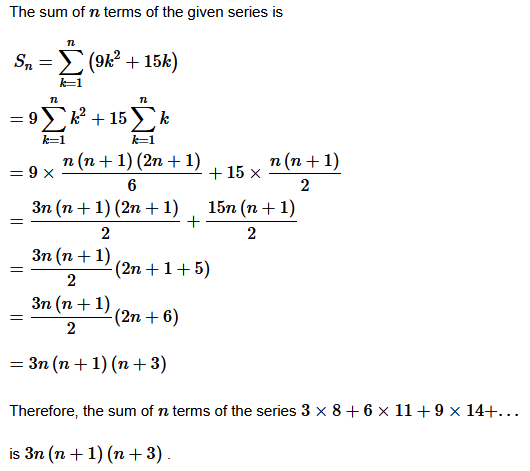Question7. Find the sum to n terms of the series 12 + (12 + 22) + (1+ 22 + 32)+…

Solution :
Given: 12 + (12 + 22) + (1+ 22 + 32)+…to nth terms

Find the sum to n terms in each of the series in Exercises 8 to 10 whose nth terms is given by

Question8. Find the sum to n terms of the series n(n+1)(n+4)

Solution :
Given:n(n+1)(n+4)

Question9. n2+2n

Solution :
Given:n2+2n

The nth term of the series is an = n2+2n

The sum of n terms of a series is given by the equationThe sum of first n terms of the given series is

Question10. Find the sum to n terms of the series  (2n−1)2

Solution :
Given:(2n−1)2

the nth term of the series is an=(2n−1)2

=4n2−4n+1The sum of n terms of a series is given by the equation.The sum of first  nth  terms of the given series is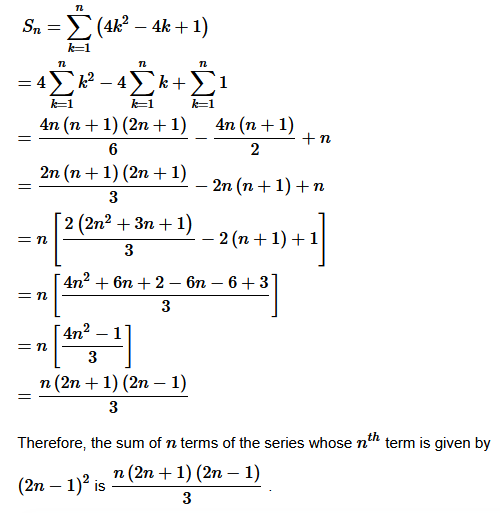Miscellaneous Exercise

Question 1. Show that the sum of (m+n)th and (m–n)th terms of an A.P. is equal to twice the mth term.

Solution :

Let the first term of the A.P. be a
and the common difference be d

.

The term of an A.P. is given by the equation

ak = a+(k−1)d

Therefore,

am+n = a+(m+n−1)d

am+n = a+(m−n−1)d

am =  a+(m−1)d

am+n + am-n = a+(m+n−1)d+a+(m−n−1)d

=2a+(m+n−1+m−n−1)d

=2a+(2m−2)d

=2a+2(m−1)d

=2[a+(m−1)d]

=2am

Therefore, the sum of (m+n)th and (m−n)th terms of an A.P. is equal to twice the mth term is proved.
Question 2. If the sum of three numbers in A.P., is 24 and their product is 440. Find the numbers.

Solution :
Let a−d , a and a+d be the three numbers in A.P. According to the conditions given in the question,

(a−d)+(a)+(a+d)=24

⇒ 3a=24

⇒a=8

(a−d) + (a) + (a+d) = 440

Substituting a=8 in the equation.

(8−d)(8)(8+d)=440

⇒(8−d)(8+d)=55

⇒64−d2 = 55

⇒d2 = 64−55

⇒d2 = 9

⇒d =±3

When d = 3 the three numbers are 5,8 and 11 When d=−3  the three numbers are 11,8 and 5

Therefore, 5,8 and 11 are the three numbers.

Question 3. Let the sum of n,2n,3n terms of an A.P. be S1,S2,S3 , respectively,  show that S3=3(S2−S1)

Solution :

Let the first term of the A.P. be a and the common difference be d

The sum of first n terms of an arithmetic progression is given by the equation…..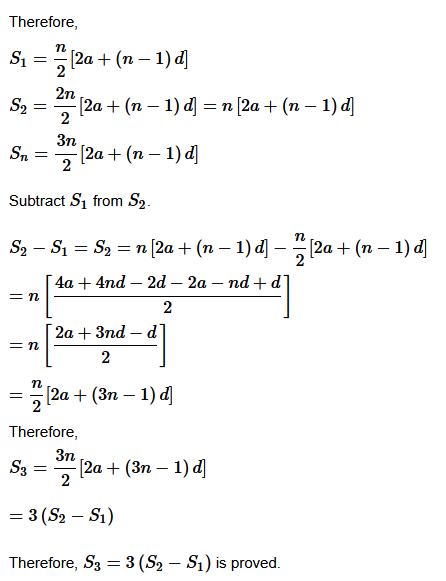Question 4. Find the sum of all numbers between 200 and 400 which are divisible by 7.

Solution :
203,210,217,…,399 are the numbers lying between 200 and 400 , which are divisible by 7 An A.P. is formed by this series.

The first term of the A.P. is a=203, the last term is an=399 and the common difference is d=7

The nth term of the A.P. is given by the equation an=a+(n−1)d

.Therefore, a+(n−1)d=399

Substitute a=203
and d=7

in the equation.

⇒203+(n−1)7=399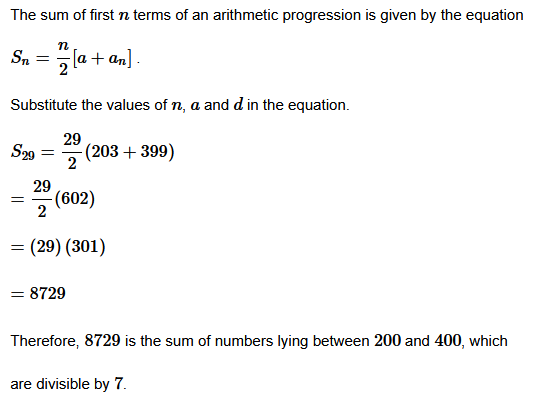The sum of first n
terms of an arithmetic progression is given by the equation

Question 5. Find the sum of integers from 1 to 100 that are divisible by 2 or 5.

Solution :
Given: A.P. which is divisible by 2
2, 4, 6, ………., 100
2,4,6,…100 are the integers lying between 1 to 100 , which are divisible by 2 . An A.P. is formed by this series.
The first term of the A.P. and the common difference is equal to 2 The nth term of the A.P. is given by the equation an = a+(n−1)d

Substitute a = 2 and d = 2 in the equation.

⇒100 = 2+(n−1)2

⇒n=50

The sum of first n terms of an arithmetic progression is given by the equation

Substitute the values of n , a and d in the equation.

2+4+6+…+100=50/2[2(2)+(50−1)(2)]

=50/2(4+98)

=(25)(102)

=2550

5,10,…100
are the integers lying between 1
to 100
, which are divisible by 5

. An A.P. is formed by this series.

The first term of the A.P. and the common difference is equal to 5 The nth
term of the A.P. is given by the equation a= a+(n−1)d

.

Substitute a=5
and d=5

in the equation.

⇒100=5+(n−1)5

⇒5n=100

⇒n=20

The sum of first n terms of an arithmetic progression is given by the equation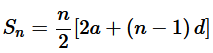Substitute the values of n, a and d  in the equation.

5+10+…+100 = 20/2[2(5)+(20−1)5]

=10[10+(19)5]

=10[10+95]

=10×105

=1050

10,20,…100
are the integers lying between 1 to 100 , which are divisible by 2 and 5

. An A.P. is formed by this series. The first term of the A.P. and the common difference is equal to 10
The nth term of the A.P. is given by the equation

an=a+(n−1)d

.Substitute a=10 and d=10

in the equation.

⇒100=10+(n−1)10

⇒100=10n

⇒n=10

The sum of first n
terms of an arithmetic progression is given by the equation.

Substitute the values of n
, a
and d

in the equation.

10+20+…+100=102[2(10)+(10−1)(10)]

=5[20+90]

=5(110)

=550

Therefore,

2250+1050−550=3050

Question 6. Find the sum of all two digit numbers which when divided by 4, yield 1 as remainder.

Solution :
Given: A.P. 13, 17, 21, ………., 97

Now

13,17,…97 are the two-digit numbers which when divided by 4
yields 1

as remainder. An A.P. is formed by this series.

The first term of the A.P. is a=13
, the last term is an=97
and the common difference is d=4

.

The nth
term of the A.P. is given by the equation an=a+(n−1)d

.

Substitute a=13
and d=4

in the equation.

⇒97=13+(n−1)4

⇒4(n−1)=84

⇒n−1=21

⇒n=22

The sum of first n
terms of an arithmetic progression is given by the equationSubstitute the values of n, a  and d  in the equation.

S22=22/2[2(13)+(22−1)(4)]

=11[26+84]

=1210

Therefore, 1210
is the sum of all two-digit numbers which when divided by 4
, yields 1 remainder

Question 7. If f is a function satisfying f (x +y) = f(x) f(y)  for all x, y ∈ N such that f(1)= 3 and  = 120, find the value of n.

Solution :
According to the given conditions in the question,

f(x+y)  = f(x)×f(y) for all x,y,∈N

f(1) = 3

Let  x = y = 1

Then,

f(1+1) = f(1+2) = f(1)f(2) = 3 × 3 =9

We can also write

f(1+1+1) = f(3) = f(1+2) = f(1)f(2) = 3×9 = 27

f(4)= f(1+4) = f(1)f(3) = 3×27 = 81

Both the first term and common ratio of f(1),f(2),f(3),…,that is 3,9,27,…,
that forms s G.P. is equal to 3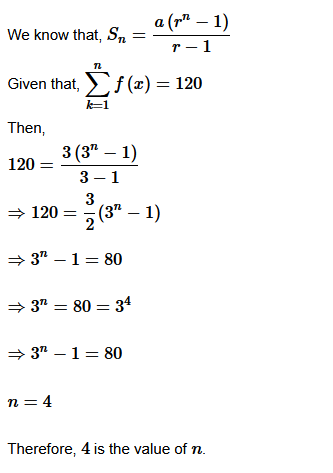Question 8. The sum of some terms of G.P. is 315 whose first term and the common ratio are 5 and 2 respectively. Find the last term and the number of terms.

Solution :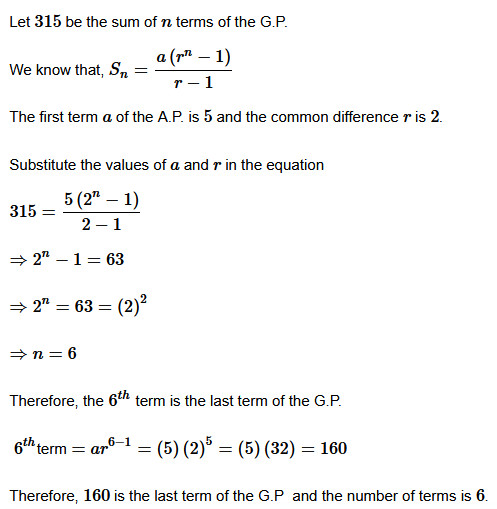Question 9. The first term of a G.P. is 1. The sum of the third term and fifth term is 90. Find common ratio of G.P.

Solution :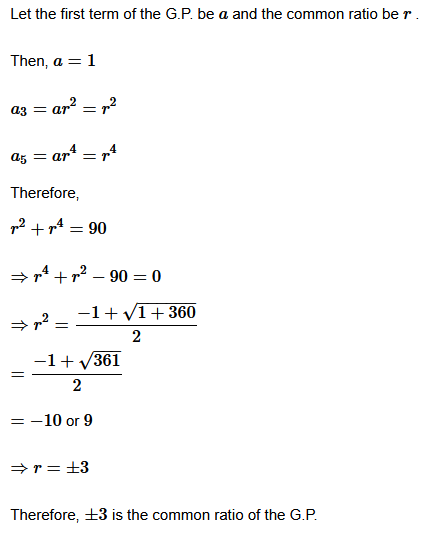Question 10. The sum of three numbers in G.P. is 56. If we subtract 1, 7, 21 from these numbers in that order, we obtain an arithmetic progression. Find the numbers.

Solution :

Let a,ar and ar2 be the three numbers in G.P. According to the conditions given in the question,

a+ar+ar2 = 56

⇒a(1+r+r2)=56  …(1)

An A.P. is formed by

a−1,ar−7,ar2−21

Therefore,

(ar−7)−(a−1) = (ar2−21)−(ar−7)b

⇒ar−a−6 = ar2−ar−14

⇒ar−2ar+a = 8

⇒ar2−ar−ar+a= 8

⇒a(r2+1−2r)=8

⇒a(r2−1)2=8

…(2)

Equating (1) and (2), we get

⇒7(r2−2r+1)=1+r+r2

⇒7r2−14r+7−1−r−r2

⇒6r2−15r+6=0

⇒6r2−12r−3r+6=0

⇒6(r−2)−3(r−2)=0

⇒(6r−3)(r−2)=0

Then,8,16 and 32 are the three numbers when r=2
and 32,16 and 8 are the numbers when r=12
.
Question 11. A G.P. consists of an even number of terms. If the sum of all the terms is 5 times the sum of terms occupying odd places, then find its common ratio.

Solution :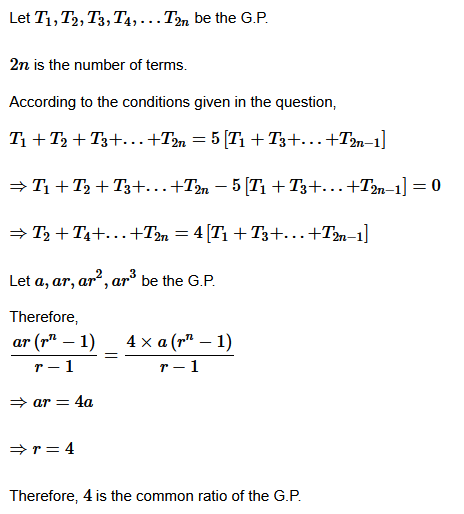Question 12. The sum of the first four terms of an A.P. is 56. The sum of the last four terms is 112. If its first term is 11, then find the number of terms.

Solution :
Let a,a+d,a+2d,a+3d…a+(n−2)d,a+(n−2)d

be the A.P.

a(a+d)+(a+2d)+(a+3d)=4a+6d

is the sum of the first four terms.

[a+(n−4)d]+[a+(n−3)d]+[a+(n−2)d]+[a+(n−1)d]=4a+(4n−10)d

is the sum of the last four terms.

According to the conditions given in the question,

4a+6d=56

It is given that a=11

, then

⇒4(11)+6d=56

⇒6d=12

⇒d=2

Therefore,

4a+(4n−10)d=112

⇒4(11)+(4n−10)2=112

⇒(4n−10)2=68

⇒4n−10=34

⇒4n=44

⇒n=11

Therefore, 11
is the number of terms of the A.P.

Question 13. If then show that a,b,c and d are in G.P.

Solution :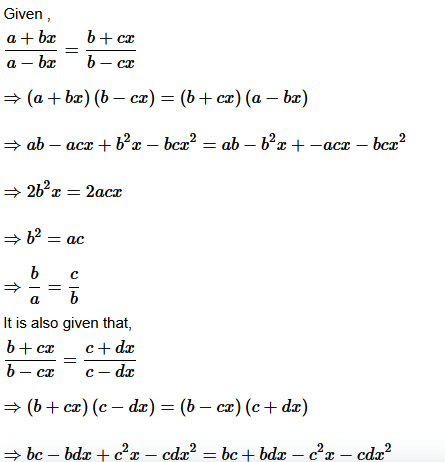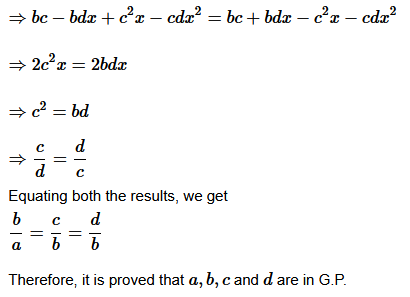Question 14. Let S be the sum, P the product and R the sum of reciprocals of n terms in a G.P. Prove that P2Rn = Sn

Solution :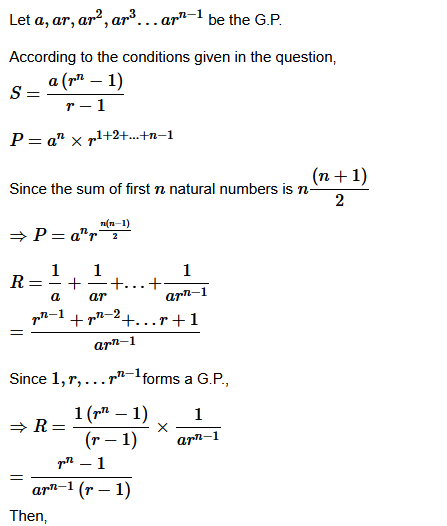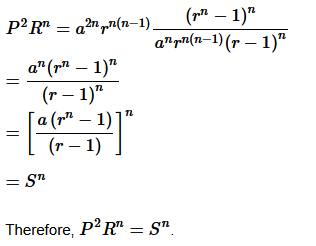Proved.
Question 15: The pth,qthand rth terms of an A.P. are a, b, c, respectively. Show that (q – r )a + (r – p )b + (p – q )c = 0

Solution :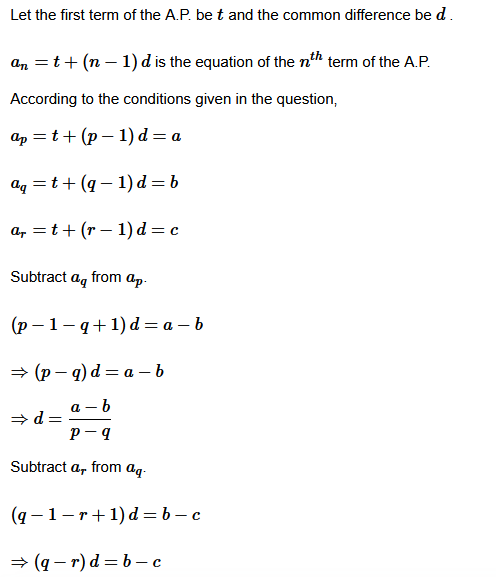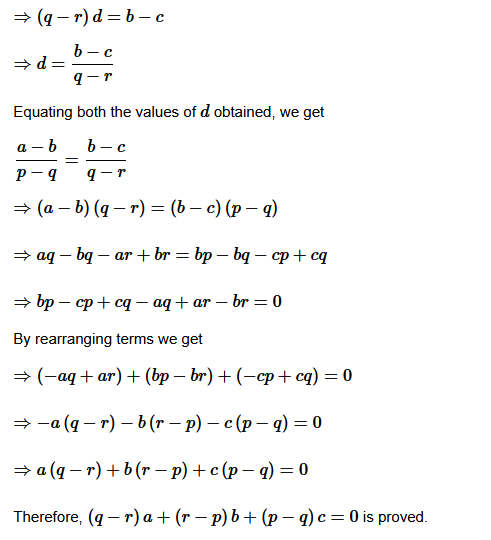Question16. If are in A.P., prove that a , b ,c are in A.P.

Solution :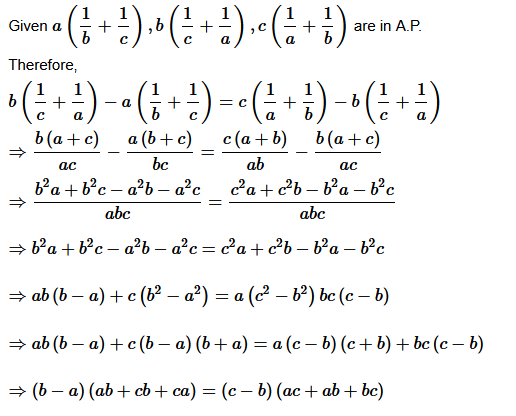Question 17. If a, b, c, d are in G.P, prove that (an + bn),(bn + cn),(cn + dn)  are in G.P.

Solution :
Given:  a, b, c, d  are in G.P.

b2 = ac

c2 = bd

To prove:

(an + bn),(b+ cn),(c+ dn)

are in G.P.

That is, (b+ cn)2 = (an + bn),(c+ dn)

Then,

L.H.S =(b+ cn)2

=b2n+2bncn+c2n

=(b2)n+2bncn+(c2)n

=(ac)n+2bncn+(bd)n

=ancn+bncn+bncn+bndn

=ancn+bncn+andn+bndn

=cn(an+bn)+dn(an+bn)

=(an+bn)(an+dn)

=

R.H.S

Therefore,

(bn+cn)2=(an+bn)(cn+dn)

Therefore, (bn+cn),(bn+cn)
and (cn+dn)
are in G.P.
Question 18.  If a and b are the roots of x2 − 3x + p = 0   and  c, d are roots of  x2−12x + q= 0 where a,b,c,d form a G.P.Prove that (q+p) : (q−p)= 17:15.

Solution :
Given: a
and b
are the roots of x2 − 3x + p =0

.Therefore,

a + b = 3 and ab=p  …(1)

We also know that c and d
are the roots of x2−12x+q=0

.Therefore,
c+d=12 and cd=q  …(2)

Also, a,b,c,d are in G.P.

Let us take a=x, b=xr,  c=xr2
and d=xr3

.

We get from (1) and (2) that,

x+xr = 3

⇒ x(1+r) =3

Also,

xr+ xr3 = 12

⇒xr+ (1+r) = 12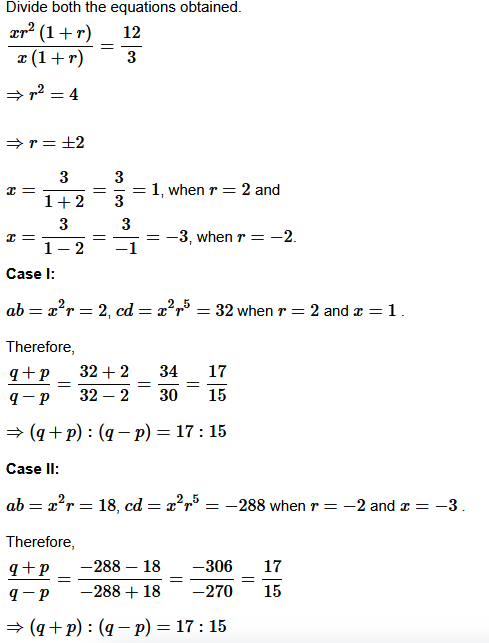Therefore, Therefore, it is proved that (q+p):(q−p) = 17:15as we obtain the same for both the cases.
Question 19. The ratio of the A.M. and G.M. of two positive numbers a and b is m : n Show that a : b =

Solution :
Given: a : b =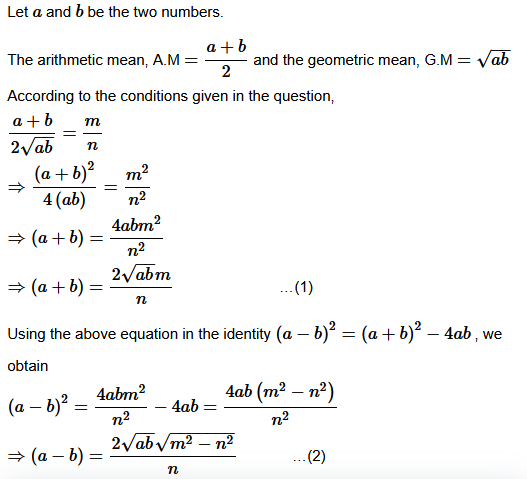By component and dividend,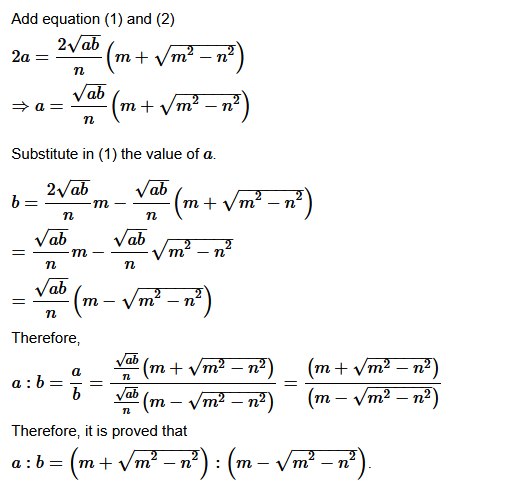Question 20. If a, b, c are in A.P.; b, c, d are in G.P. and 1/c,1/d,1/e are in A.P. prove that a, c, e are in G.P.

Solution :
Since,

Given a,b,c are in A.P.Therefore, b−a=c−b are in A.P.
⇒ 2b =  a + c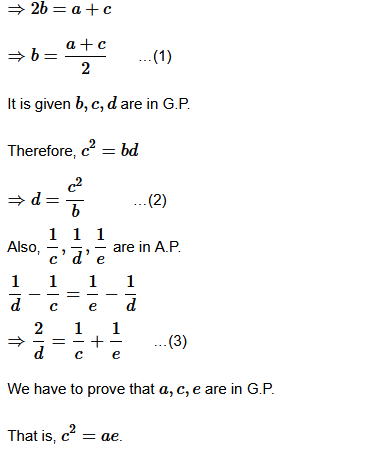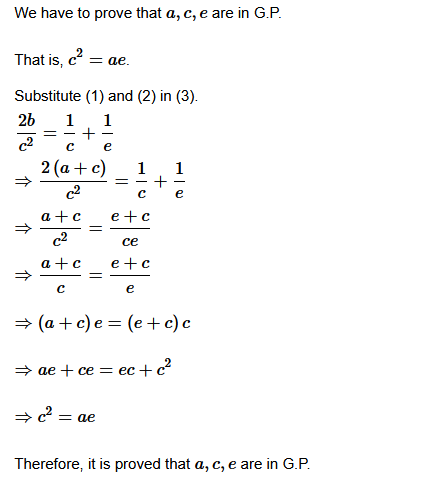Question 21. Find the sum of the following series up to n terms:
(i) 5 + 55 + 555 + ……….
(ii) .6 + .66 + .666 + ………….

Solution :
(i) sn = 5 + 55 + 555 + ………. up to n terms
= 5/9 [1 + 11 + 111 + ………. up to n terms]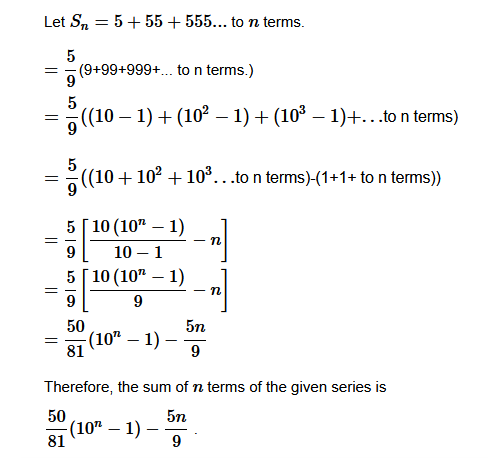(ii) sn = .6 + .66 + .666 + …………. up to n terms
= 6 [.1 + .11 + .111 + ………. up to n terms]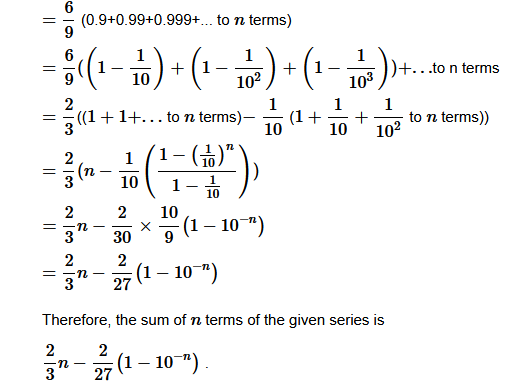Question 22. Find the 20th term of the series 2 × 4 + 4 × 6 + 6 × 8 + … + n terms.

Solution :
Given: 2 × 4 + 4 × 6 + 6 × 8 + … + n terms
Therefore the nth term an=2n×(2n+2)=4n2+4n

Then,

a20 =4(20)2 + 4(20)

=4(400)+80

=1600+80

=1680

Therefore, 1680
is the 20th

Question23. Find the sum of the first n terms of the series: 3 + 7 + 13 + 21 + 21 + ……….
Solution :
Given: 3 + 7 + 13 + 21 + 21 + ……………….(i)
Also 3+7+13+21+31+…

is the given series.

S=3+7+13+21+31+…+an−1 + an

S=3+7+13+21+…+ an−2 + an−1 + an ……….(ii)
Subtracting eq. (ii) from eq. (i),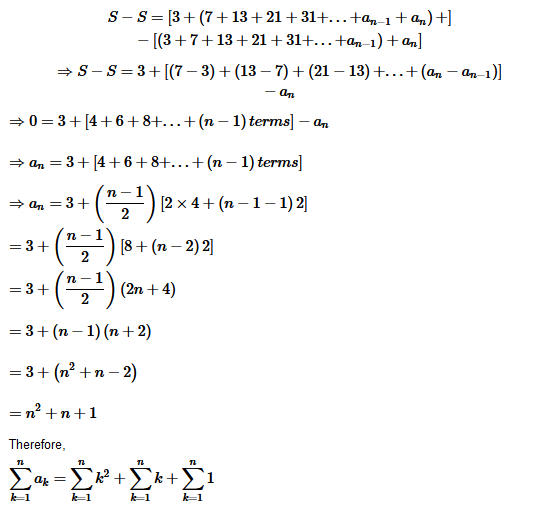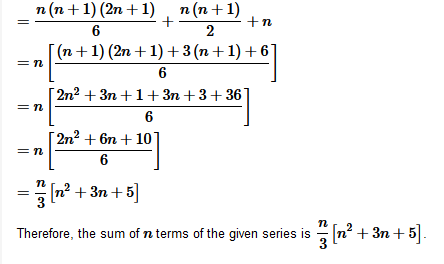Question 24.  If S1,S2,S3 are the sum of first n natural numbers, their squares and their  cubes, respectively, show that 9S22= S3 (1+8S1)

Solution :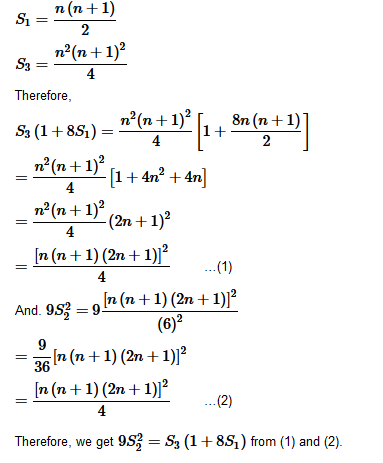Question 25. Find the sum of the following series up to n terms: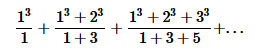Solution :
Given:up to nth terms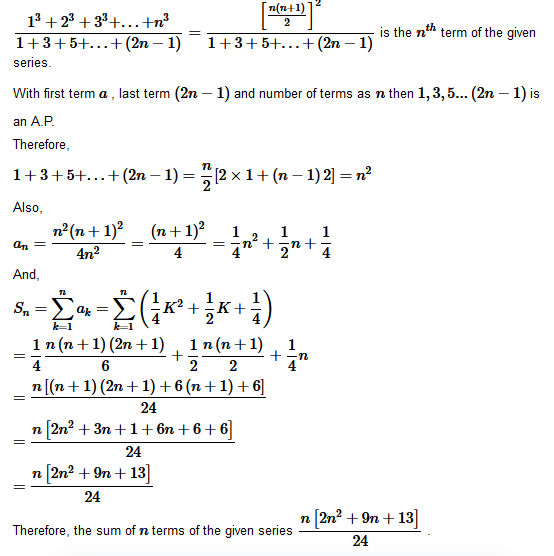Question 26. Show that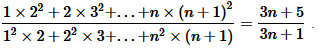Solution :
Given: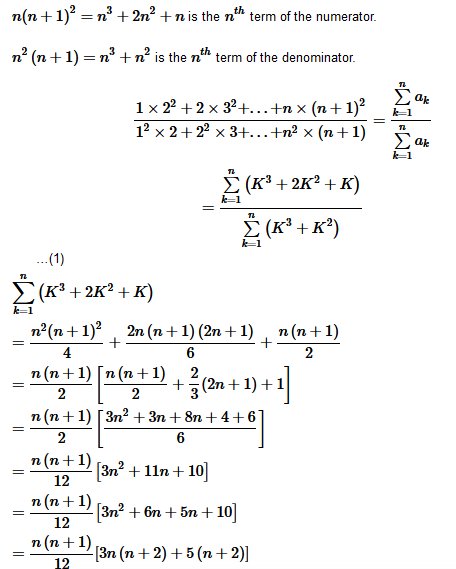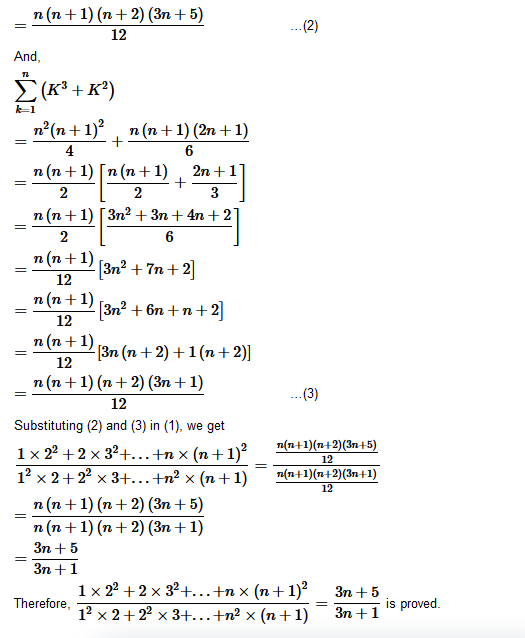Question 27. A farmer buys a used tractor for Rs. 12000. He pays Rs. 6000 cash and agrees to pay the balance in annual installments of Rs. 500 plus 12% interest on the unpaid amount. How much will the tractor cost him?

Solution :
It is given that Rs.6000

is paid in cash by the farmer.

Therefore, the unpaid amount is given by

Rs.12000−Rs.6000 =  Rs.6000

According to the conditions given in the question, the interest to be paid annually by the farmer is

12 of 6000 , 12 of 5500 , 12 of 5000…12 of 500

Therefore, the total interest to be paid by the farmer

=12 of 6000+12 of 5500+12 of 5000+…+12 of 500

=12 of (6000+5500+5000+…+500)

=12 of (500+1000+1500+…+6000)

With both the first term and common difference equal to 500
, the series 500,1000,1500…6000 is an A.P.

Let n

be the number of terms of the A.P.

Therefore,

6000=500+(n−1)500

⇒1+(n−1)=12

⇒n=12

Therefore, the sum of the given A.P.

=122[2(500)+(12−1)(500)]

=6[1000+5500]

=6(6500)

=39000

Therefore, the total interest to be paid by the farmer

=12 of (500+1000+1500+…+6000)

=12 of Rs.39000

= Rs.4680

Therefore, the total cost of tractor

= (Rs.12000+ Rs.4680 )

= Rs.16680

Therefore, the total cost of the tractor is Rs.16680
Question 28. Shams had Ali buys a scooter for Rs. 22000. He pays Rs. 4000 cash and agrees to pay the balance in annual installment of Rs. 1000 plus 10% interest on the unpaid amount. How much will the scooter cost him?

Solution :
It is given that for Rs.22000 Shamshad Ali buys a scooter and Rs.4000
is paid in cash. Therefore, the unpaid amount is given by

Rs.22000−Rs.4000 = Rs.18000

According to the conditions given in the question, the interest to be paid annuallyis

10 of 18000 , 10 of 17000, 10 of 16000…10 of 1000

Therefore, the total interest to be paid by the farmer

=10 of 18000+10 of 17000+1 of 16000+…+10 of 1000

=10 of (18000+17000+16000+…+1000)

=10 of (1000+2000+3000+…+18000)

With both the first term and common difference equal to 1000
, the series 1000,2000,3000…18000 is an A.P.
Let n be the number of terms of the A.P.

Therefore,

18000=1000+(n−1)1000

⇒1+(n−1)=18

⇒n=18

Therefore, the sum of the given A.P.

=18/2 [2(1000)+(18−1)(1000)]

=9[2000+17000]

=9(19000)

=171000

Therefore, the total interest to be paid

=10 of (18000+17000+16000+…+1000)

=10 of  Rs.171000

=
Rs.17100

Therefore, the total cost of scooter

=
(Rs.22000 + Rs.17100)

=
Rs.39100

Therefore, the total cost of the scooter is Rs.39100
Question 29. A person writes a letter to four of his friends. He asks each one of them to copy the letter and mail to four different persons with instruction that they move the chain similarly. Assuming that the chain is not broken and that it costs 50 paise to mail one letter. Find the amount spent on the postage when 8th set of letter is mailed.

Solution :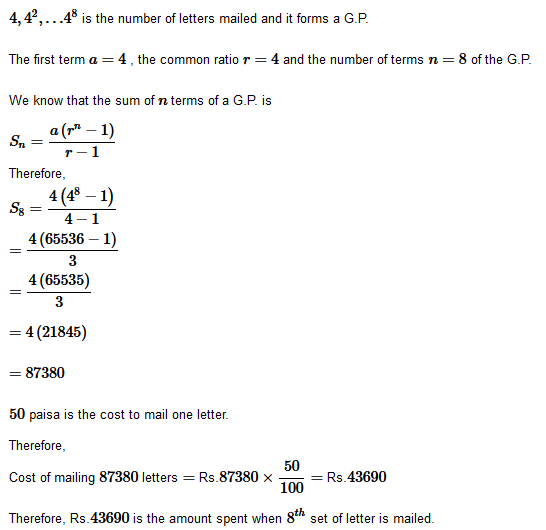Question 30. A man deposited Rs. 10000 in a bank at the rate of 5% simple interest annually. Find the amount in 15th year since he deposited the amount and also calculate the total amount after 20 years.

Solution :
Rs.10000 is deposited by the man in a bank at the rate of 5 simple interest annually

=5/ 100 × Rs.10000 = Rs.500

Therefore,
10000+500+500+…+500 is the interest in 15th
year. (500 is 14 added times)

Therefore, the amount in 15th
year = Rs.10000+14 × Rs.500

= Rs.10000 + Rs.7000

= Rs.17000

Rs.10000+500+500+…+500
is the amount after 20 years. (500 is 20 added times)

Therefore, the amount after 20

years = Rs.10000+20 × Rs.500

= Rs.10000 + Rs.10000

=
Rs.20000

The total amount after 20 years is Rs.20000
Question 31. A manufacturer reckons that the value of a machine, which cost him Rs. 15625 will depreciate each year by 20%. Find the estimated value at the end of 5 years.

Solution :
The cost of the machine is Rs.15625.

Every year machine depreciates by 20.

Therefore, 80 of the original cost ,i.e., 45 of the original cost is its value after every year.

Therefore, the value at the end of 5 years

=15626×45×45×…×45

=5×1024

=5120

Therefore, Rs.5120
is the value of the machine at the end of 5
years.
Question 32. 150 workers were engaged to finish a job in a certain number of boys. 4 workers dropped out on second day, 4 more workers dropped out on third day and so on. It took 8 more days to finish the work find the number of days in which the work was completed.

Solution :
Let the number of days in which 150 workers finish the work be x

.According to the conditions given in the question,

150x=150+146+142+…(x+8)

terms

With first term a=146, common difference d=−4 and number of turns as (x+8) , the series 150+146+142+…(x+8)

terms is an A.P.

⇒150x=(x+8)/2[2(150)+(x+8−1)(−4)]

⇒150x=(x+8)[150+(x+7)(−2)]

⇒150x=(x+8)(150−2x−14)

⇒150x=(x+8)(136−2x)

⇒75x=(x+8)(68−x)

⇒75x=68x−x2+544−8x

⇒x2+75x−60x−544=0

⇒x2+15x−544=0

⇒x2+32x−17x−544=0

⇒x(x+32)−17(x+32)=0

⇒(x−17)(x+32)=0

⇒x=17
or x=−32

We know that x cannot be negative.

So, x=17

Therefore, 17
is the number of days in which the work was completed. Then the required number of days =(17+8)=25

Get 30% off your first purchase!

X
error: Content is protected !!
Scroll to Top